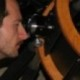## A quite effective QCD theory

As far as my path toward understanding of QCD is concerned, I have found a quite interesting effective theory to work with that is somewhat similar to Yukawa theory. Hideki Yukawa turns out to be more in depth in his hindsight than expected.Indeed, I have already showed as the potential in infrared Yang-Mills theory is an infinite sum of weighted Yukawa potentials with the range, at each order, decided through a mass formula for glueballs that can be written down as$m_n=(2n+1)\frac{\pi}{2K(i)}\sqrt{\sigma}$

being$\sigma$ the string tension, an experimental parameter generally taken to be$(440 MeV)^2$, and$K(i)$ is an elliptic integral, just a number.

The most intriguing aspect of all this treatment is that an effective infrared QCD can be obtained through a scalar field. I am about to finish a paper with a calculation of the width of the$\sigma$ resonance, a critical parameter for our understanding of low energy QCD. Here I put the generating functional if someone is interested in doing a similar calculation (time is rescaled as$t\rightarrow\sqrt{N}gt$)$Z[\eta,\bar\eta,j] \approx\exp\left\{i\int d^4x\sum_q \frac{\delta}{i\delta\bar\eta_q(x)}\frac{\lambda^a}{2\sqrt{N}}\gamma_i\eta_i^a\frac{\delta}{i\delta j_\phi(x)}\frac{i\delta}{\delta\eta_q(x)}\right\} \times$$\exp\left\{-\frac{i}{Ng^2}\int d^4xd^4y\sum_q\bar\eta_q(x)S_q(x-y)\eta_q(y)\right\}\times$$\exp\left\{\frac{i}{2}(N^2-1)\int d^4xd^4y j_\phi(x)\Delta(x-y)j_\phi(y)\right\}.$

As always,$S_q(x-y)$ is the free Dirac propagator for the given quark$q=u,d,s,\ldots$ and$\Delta(x-y)$ is the gluon propagator that I have discussed in depth in my preceding posts. People seriously interested about this matter should read my works (here and here).

For a physical understanding of this you have to wait my next posting on arxiv. Anyhow, anybody can spend some time to manage this theory to exploit its working and its fallacies. My hope is that, anytime I post such information on my blog, I help the community to have an anticipation of possible new ways to see an old problem with a lot of prejudices well grounded.

### 2 Responses to A quite effective QCD theory

1.dorigo says:

Marco, indeed by putting online this information you pay a great service to the community. I cannot grasp much of the details, but I am sure there are carefully observing eyes out there. Transparency in physics research is an objective to pursue with more momentum than primacy in publishing.

Cheers,
T.

2.mfrasca says:

Hi Tommaso,

I greatly appreciate your comment. Of course, our main aim is to get our ideas published on archival journals but, to let them known with such a powerful media like a blog can be a way to have some relevant criticisms and comments that can improve or show a way to see how bad the idea is. I agree with your comment about transparency in a moment where physics is in a kind of static situation where experiments do not seem to help too much (even if CDF collaboration can be the first kind of light after many years of dark :-))

Ciao,

Marco

This site uses Akismet to reduce spam. Learn how your comment data is processed.